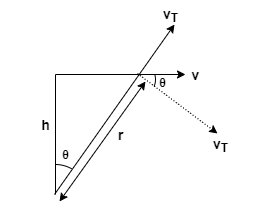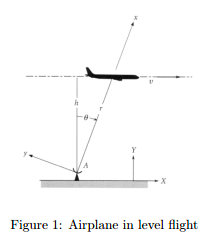# Problem:  An airplane in level flight at an altitude h and a uniform speed u passes directly over a radar tracking station A. Calculate the angular velocity theta and angular acceleration of the radar antenna theta as well as the rate r at which the airplane is moving away from the antenna. Attach the inertial frame of reference to the ground and assume non - rotating earth. Attach the moving frame to the antenna, with the x-axis pointing always from the antenna towards the airplane.

###### FREE Expert Solution

Angular velocity:

$\overline{)\stackrel{\mathbf{.}}{\mathbf{\theta }}{\mathbf{=}}\frac{{\mathbf{v}}_{\mathbf{T}}}{\mathbf{r}}}$, where $\stackrel{\mathbf{.}}{\mathbf{\theta }}$ is the differential of the angular displacement θ with respect to time, vT is tangential velocity, and r is the radius.

Let's consider the diagram:From the figure:

94% (120 ratings)###### Problem Details

An airplane in level flight at an altitude h and a uniform speed u passes directly over a radar tracking station A. Calculate the angular velocity theta and angular acceleration of the radar antenna theta as well as the rate r at which the airplane is moving away from the antenna. Attach the inertial frame of reference to the ground and assume non - rotating earth. Attach the moving frame to the antenna, with the x-axis pointing always from the antenna towards the airplane.Frequently Asked Questions

What scientific concept do you need to know in order to solve this problem?

Our tutors have indicated that to solve this problem you will need to apply the Rotational Velocity & Acceleration concept. You can view video lessons to learn Rotational Velocity & Acceleration. Or if you need more Rotational Velocity & Acceleration practice, you can also practice Rotational Velocity & Acceleration practice problems.

What professor is this problem relevant for?

Based on our data, we think this problem is relevant for Professor Jerousek's class at UCF.